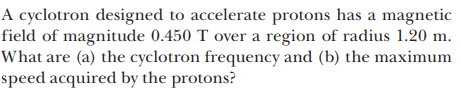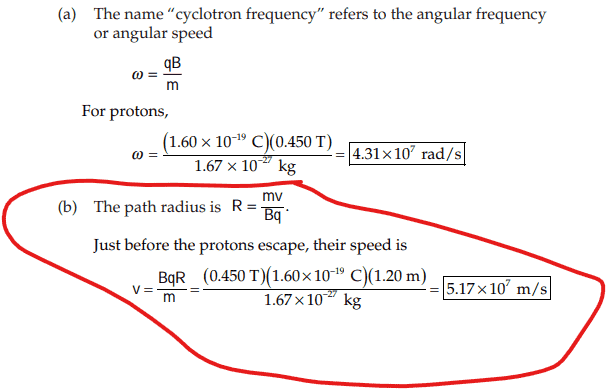# Max speed of proton in cyclotron

Callumnc1
Homework Statement:
Relevant Equations:
For this problem,The solution is,However, I don't understand why they say just before the proton escapes? Are they assuming that the B-field is only at a portion of region which means that only half or so of the circular path is within the B-field so only half of the path have a magnetic centripetal force?

If the B-field is only there for a portion of the protons path, how do they know that max speed is reached?

Many thanks!

Homework Helper
Gold Member
The say "just before the proton escapes" to give you indirectly a numerical value. Which of the two values in the statement of the problem do you think that is?

•Callumnc1
Callumnc1
The say "just before the proton escapes" to give you indirectly a numerical value. Which of the two values in the statement of the problem do you think that is?
The max speed is ##5.17 \times 10^7 \frac {m}{s}##?

Homework Helper
Gold Member
The max speed is ##5.17 \times 10^7 \frac {m}{s}##?
I said the "in statement of the problem", not in the solution that follows it.

•Callumnc1
Callumnc1
I said the "in statement of the problem", not in the solution that follows it.
Oh I see - thanks @kuruman!

It means max speed of proton over region of radius 1.20 m with B-field strength of 0.450 T.

EDIT: However, I still don't see how the proton would escape since theoretically it should continue moving in the circle of radius 1.20 m as the magnetic force provides the centripetal force. What would cause it to leave the B-field?

Homework Helper
Gold Member
Protons in a cyclotron are accelerated. This means that the radius of the orbit increases until they escape. Look it up here to see how it's done.

•Callumnc1
Callumnc1
Protons in a cyclotron are accelerated. This means that the radius of the orbit increases until they escape. Look it up here to see how it's done.
Thanks @kuruman , will do!

Callumnc1
Thanks @kuruman , will do!
Ah so understanding the cyclotron principle I see now that you don't have to calculate the electric force on the protons going from each dee as that info can be gauged from the magnetic field strength and cyclon frequency that does not depend on radius.